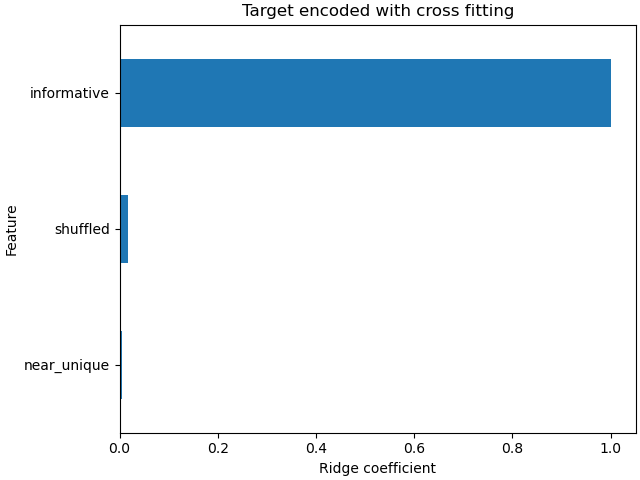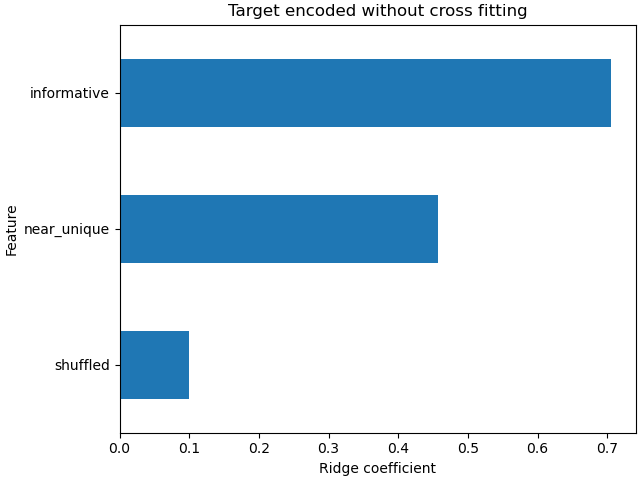# Target Encoder’s Internal Cross fitting¶

The TargetEncoder replaces each category of a categorical feature with the shrunk mean of the target variable for that category. This method is useful in cases where there is a strong relationship between the categorical feature and the target. To prevent overfitting, TargetEncoder.fit_transform uses an internal cross fitting scheme to encode the training data to be used by a downstream model. This scheme involves splitting the data into k folds and encoding each fold using the encodings learnt using the other k-1 folds. In this example, we demonstrate the importance of the cross fitting procedure to prevent overfitting.

## Create Synthetic Dataset¶

For this example, we build a dataset with three categorical features:

• an informative feature with medium cardinality (“informative”)

• an uninformative feature with medium cardinality (“shuffled”)

• an uninformative feature with high cardinality (“near_unique”)

First, we generate the informative feature:

import numpy as np

from sklearn.preprocessing import KBinsDiscretizer

n_samples = 50_000

rng = np.random.RandomState(42)
y = rng.randn(n_samples)
noise = 0.5 * rng.randn(n_samples)
n_categories = 100

kbins = KBinsDiscretizer(
n_bins=n_categories,
encode="ordinal",
strategy="uniform",
random_state=rng,
subsample=None,
)
X_informative = kbins.fit_transform((y + noise).reshape(-1, 1))

# Remove the linear relationship between y and the bin index by permuting the
# values of X_informative:
permuted_categories = rng.permutation(n_categories)
X_informative = permuted_categories[X_informative.astype(np.int32)]


The uninformative feature with medium cardinality is generated by permuting the informative feature and removing the relationship with the target:

X_shuffled = rng.permutation(X_informative)


The uninformative feature with high cardinality is generated so that it is independent of the target variable. We will show that target encoding without cross fitting will cause catastrophic overfitting for the downstream regressor. These high cardinality features are basically unique identifiers for samples which should generally be removed from machine learning datasets. In this example, we generate them to show how TargetEncoder’s default cross fitting behavior mitigates the overfitting issue automatically.

X_near_unique_categories = rng.choice(
int(0.9 * n_samples), size=n_samples, replace=True
).reshape(-1, 1)


Finally, we assemble the dataset and perform a train test split:

import pandas as pd

from sklearn.model_selection import train_test_split

X = pd.DataFrame(
np.concatenate(
[X_informative, X_shuffled, X_near_unique_categories],
axis=1,
),
columns=["informative", "shuffled", "near_unique"],
)
X_train, X_test, y_train, y_test = train_test_split(X, y, random_state=0)


## Training a Ridge Regressor¶

In this section, we train a ridge regressor on the dataset with and without encoding and explore the influence of target encoder with and without the internal cross fitting. First, we see the Ridge model trained on the raw features will have low performance. This is because we permuted the order of the informative feature meaning X_informative is not informative when raw:

import sklearn
from sklearn.linear_model import Ridge

# Configure transformers to always output DataFrames
sklearn.set_config(transform_output="pandas")

ridge = Ridge(alpha=1e-6, solver="lsqr", fit_intercept=False)

raw_model = ridge.fit(X_train, y_train)
print("Raw Model score on training set: ", raw_model.score(X_train, y_train))
print("Raw Model score on test set: ", raw_model.score(X_test, y_test))

Raw Model score on training set:  0.0049896314219657345
Raw Model score on test set:  0.00457762158159003


Next, we create a pipeline with the target encoder and ridge model. The pipeline uses TargetEncoder.fit_transform which uses cross fitting. We see that the model fits the data well and generalizes to the test set:

from sklearn.pipeline import make_pipeline
from sklearn.preprocessing import TargetEncoder

model_with_cf = make_pipeline(TargetEncoder(random_state=0), ridge)
model_with_cf.fit(X_train, y_train)
print("Model with CF on train set: ", model_with_cf.score(X_train, y_train))
print("Model with CF on test set: ", model_with_cf.score(X_test, y_test))

Model with CF on train set:  0.8000184677460285
Model with CF on test set:  0.7927845601690948


The coefficients of the linear model shows that most of the weight is on the feature at column index 0, which is the informative feature

import matplotlib.pyplot as plt
import pandas as pd

plt.rcParams["figure.constrained_layout.use"] = True

coefs_cf = pd.Series(
model_with_cf[-1].coef_, index=model_with_cf[-1].feature_names_in_
).sort_values()
ax = coefs_cf.plot(kind="barh")
_ = ax.set(
title="Target encoded with cross fitting",
xlabel="Ridge coefficient",
ylabel="Feature",
)While TargetEncoder.fit_transform uses an internal cross fitting scheme to learn encodings for the training set, TargetEncoder.transform itself does not. It uses the complete training set to learn encodings and to transform the categorical features. Thus, we can use TargetEncoder.fit followed by TargetEncoder.transform to disable the cross fitting. This encoding is then passed to the ridge model.

target_encoder = TargetEncoder(random_state=0)
target_encoder.fit(X_train, y_train)
X_train_no_cf_encoding = target_encoder.transform(X_train)
X_test_no_cf_encoding = target_encoder.transform(X_test)

model_no_cf = ridge.fit(X_train_no_cf_encoding, y_train)


We evaluate the model that did not use cross fitting when encoding and see that it overfits:

print(
"Model without CF on training set: ",
model_no_cf.score(X_train_no_cf_encoding, y_train),
)
print(
"Model without CF on test set: ",
model_no_cf.score(
X_test_no_cf_encoding,
y_test,
),
)

Model without CF on training set:  0.858486250088675
Model without CF on test set:  0.6338211367110066


The ridge model overfits because it assigns much more weight to the uninformative extremely high cardinality (“near_unique”) and medium cardinality (“shuffled”) features than when the model used cross fitting to encode the features.

coefs_no_cf = pd.Series(
model_no_cf.coef_, index=model_no_cf.feature_names_in_
).sort_values()
ax = coefs_no_cf.plot(kind="barh")
_ = ax.set(
title="Target encoded without cross fitting",
xlabel="Ridge coefficient",
ylabel="Feature",
)## Conclusion¶

This example demonstrates the importance of TargetEncoder’s internal cross fitting. It is important to use TargetEncoder.fit_transform to encode training data before passing it to a machine learning model. When a TargetEncoder is a part of a Pipeline and the pipeline is fitted, the pipeline will correctly call TargetEncoder.fit_transform and use cross fitting when encoding the training data.

Total running time of the script: (0 minutes 0.283 seconds)

Gallery generated by Sphinx-Gallery